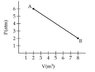# Thermodynamics and waves problems

• kelvin56484984

## Homework Statement

1.
A gas expands as shown in the graph. If the heat taken in during this process is 1.02 × 10^6 J, the change in internal energy of the gas (in J) is
2.
One mole of an ideal gas is held at a constant pressure of 1 atm. Find the change in volume if the temperature changes by 50°C.
3.
Two harmonic waves traveling in opposite directions interfere to produce a standing wave described by y = 2 sin (π x) cos (3π t) where x is in m and t is in s. What is the distance (in m) between two adjacent antinodes?

pV=nRT
W= -p(V2-V1)
E= Q+W

## The Attempt at a Solution

1.
E=Q+W
E=1.02*10^6+[-p(V2-V1)]
E=1.02*10^6-(6-2)(8-2)
It seems incorrect

2.
pV=nRT
1*V=1*8.31*(50)
V=416 m^3
3.
k=2pi/λ
pi=2pi/λ
λ=2
pi=[3pi]/λ
λ=3
the distance is 3-2=1

#### Attachments

•gas.JPG
6.2 KB · Views: 494

## Homework Statement

1.
A gas expands as shown in the graph. If the heat taken in during this process is 1.02 × 10^6 J, the change in internal energy of the gas (in J) is

## Homework Equations

W= -p(V2-V1)
Incorrect. p is not constant with V.
E= Q+W
Right. Get the right W.

## Homework Statement

1.
A gas expands as shown in the graph. If the heat taken in during this process is 1.02 × 10^6 J, the change in internal energy of the gas (in J) is
2.
One mole of an ideal gas is held at a constant pressure of 1 atm. Find the change in volume if the temperature changes by 50°C.
3.
Two harmonic waves traveling in opposite directions interfere to produce a standing wave described by y = 2 sin (π x) cos (3π t) where x is in m and t is in s. What is the distance (in m) between two adjacent antinodes?

pV=nRT
W= -p(V2-V1)
E= Q+W

## The Attempt at a Solution

1.
E=Q+W
E=1.02*10^6+[-p(V2-V1)]
E=1.02*10^6-(6-2)(8-2)
It seems incorrect

2.
pV=nRT
1*V=1*8.31*(50)
V=416 m^3
3.
k=2pi/λ
pi=2pi/λ
λ=2
pi=[3pi]/λ
λ=3
the distance is 3-2=1
When you have three different HW problems, the recommended procedure is to start a thread for each problem. It is less confusing that way.